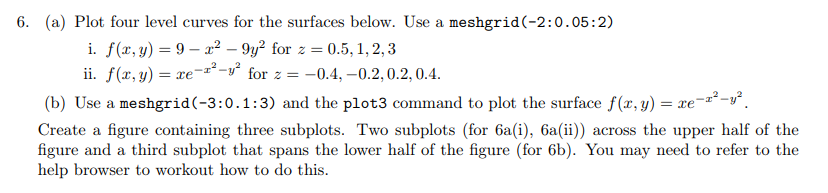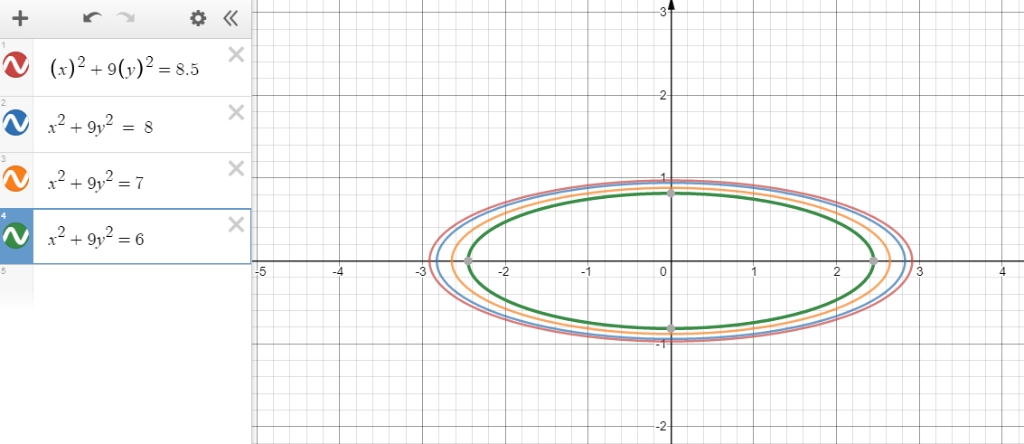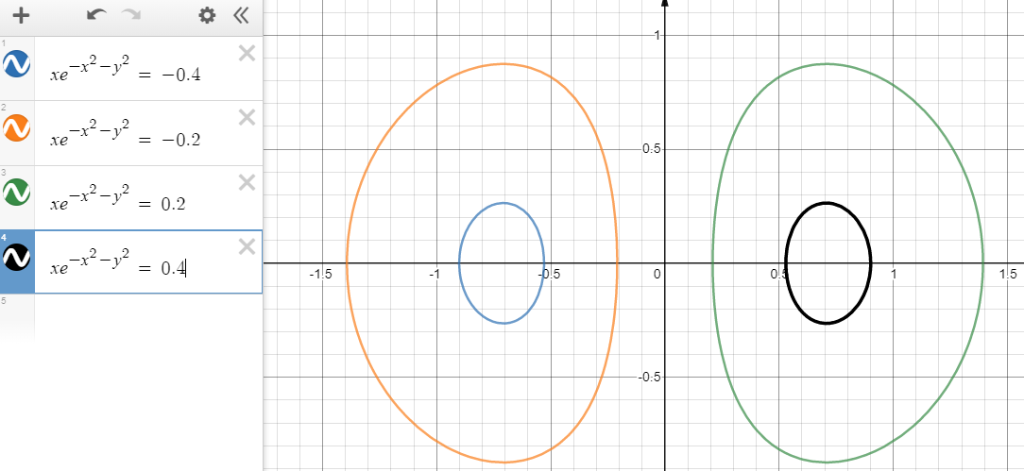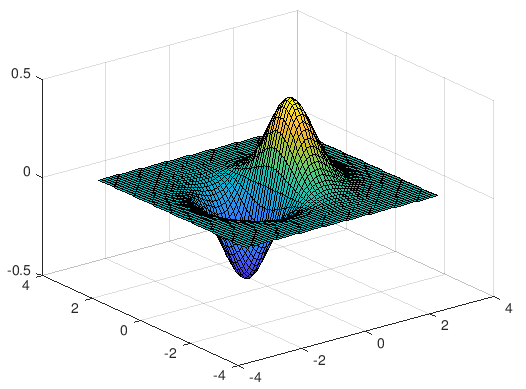# 6. (a) Plot four level curves for the surfaces below. Use a meshgrid(-2:0.05:2) i. f(z, y)-9-r2-9y2 for z = 0.5, 1, 2,...6. (a) Plot four level curves for the surfaces below. Use a meshgrid(-2:0.05:2) i. f(z, y)-9-r2-9y2 for z = 0.5, 1, 2, 3 ii. f(x, y) - for0.4,-0.2,0.2,0.4. (b) Use a meshgrid(-3:0.1:3) and the plot3 command to plot the surface f(, y) Create a figure containing three subplots. Two subplots (for 6a(i), 6a(ii)) across the upper half of the figure and a third subplot that spans the lower half of the figure (for 6b). You may need to refer to the help browser to workout how to do this.b) Plot of the surface.##### Add Answer of: 6. (a) Plot four level curves for the surfaces below. Use a meshgrid(-2:0.05:2) i. f(z, y)-9-r2-9y2 for z = 0.5, 1, 2,...
More Homework Help Questions Additional questions in this topic.